Test: Power Systems- 4

# Test: Power Systems- 4

Test Description

## 10 Questions MCQ Test GATE Electrical Engineering (EE) 2023 Mock Test Series | Test: Power Systems- 4

Test: Power Systems- 4 for Electrical Engineering (EE) 2023 is part of GATE Electrical Engineering (EE) 2023 Mock Test Series preparation. The Test: Power Systems- 4 questions and answers have been prepared according to the Electrical Engineering (EE) exam syllabus.The Test: Power Systems- 4 MCQs are made for Electrical Engineering (EE) 2023 Exam. Find important definitions, questions, notes, meanings, examples, exercises, MCQs and online tests for Test: Power Systems- 4 below.
Solutions of Test: Power Systems- 4 questions in English are available as part of our GATE Electrical Engineering (EE) 2023 Mock Test Series for Electrical Engineering (EE) & Test: Power Systems- 4 solutions in Hindi for GATE Electrical Engineering (EE) 2023 Mock Test Series course. Download more important topics, notes, lectures and mock test series for Electrical Engineering (EE) Exam by signing up for free. Attempt Test: Power Systems- 4 | 10 questions in 30 minutes | Mock test for Electrical Engineering (EE) preparation | Free important questions MCQ to study GATE Electrical Engineering (EE) 2023 Mock Test Series for Electrical Engineering (EE) Exam | Download free PDF with solutions
 1 Crore+ students have signed up on EduRev. Have you?
Test: Power Systems- 4 - Question 1

### Consider the following statements: The VAR injection in a power system is obtained by: 1. Tap changing transformers 2. Synchronous condensers 3. Capacitor and inductor banks 4. Inductor banks Which of the following statements given above are correct?

Detailed Solution for Test: Power Systems- 4 - Question 1

The purpose of a tap changer is to regulate the output voltage of a transformer. It does this by altering the number of turns in one winding and thereby changing the turn’s ratio of the transformer.

Synchronous condensers and capacitor banks are used to inject leading VAR into system where as inductor banks are used to inject lagging VAR into system.

*Answer can only contain numeric values
Test: Power Systems- 4 - Question 2

### An induction motor operating at 0.8 pf lag consuming 500 kW. A zero real power consuming synchronous motors is connected across the induction motor raise the power factor to unity. The reactive power drawn by synchronous motor is___ (kVAR)

Detailed Solution for Test: Power Systems- 4 - Question 2

P = 500 kW, Power factor = 0.8

KVA = 500/0.8 = 625 KVA

Reactive power drawn = 625 × 0.6 = 375 kVAR

To rise the power factor to unity, the total kVAR drawn by induction motor should be supplied by synchronous motor. KVAR drawn by synchronous motor is 375 kVAR.

Test: Power Systems- 4 - Question 3

### A 10 kW induction motor initially operating on a power factor of 0.5 lagging. If power factor is improved to 0.866 lagging than what is the value of kVAr raised

Detailed Solution for Test: Power Systems- 4 - Question 3

Raised KV Ar = 10(tan600 − tan300) =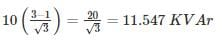*Answer can only contain numeric values
Test: Power Systems- 4 - Question 4

Shunt capacitor are used to raise the power factor of the load of 150 kW from 0.75 lag to unity, the supply being 3 – phase at 11 kV. In star bank the capacitance will be – (in μF)

Detailed Solution for Test: Power Systems- 4 - Question 4

KV AR = P tan ϕ
= 150 × 103 × 0.88 = 132.287 × 103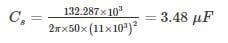Test: Power Systems- 4 - Question 5

At an Industrial substation with a 4 MW load a capacitor of 2.5 MVAR is installed to maintain the load power factor at 0.97 lag. If the capacitor goes out of service, the load power factor becomes –

Detailed Solution for Test: Power Systems- 4 - Question 5

PL = 4MW
QC = 2.5MV AR

Pf = 0.97 lag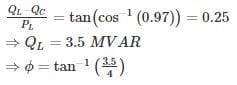⇒ Power factor =cosϕ=0.75lag

*Answer can only contain numeric values
Test: Power Systems- 4 - Question 6

A 270 KV transmission line has the following line constants A = 0.85∠5° ; B = 200∠75°. What is the power that can be received if the voltage profile at each end is maintained at 270 KV (in MW) –

Detailed Solution for Test: Power Systems- 4 - Question 6

Given |VS|= |VR| = 270 KV, α = 5°, β = 75°

since the power is received at unity power factor QR = 0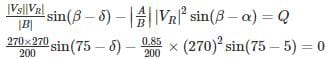⇒ δ = 22°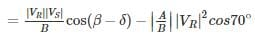= 219.6 – 105.96 = 113.64 MW

*Answer can only contain numeric values
Test: Power Systems- 4 - Question 7

A single phase 50 Hz motor takes 20 A at 0.75 power factor lagging from a 220 V sinusoidal supply. The capacitance required to be connected in parallel to raise the power factor to 0.9 lagging is ______ (in μF)

Detailed Solution for Test: Power Systems- 4 - Question 7

I1 = 20A, f = 50 Hz
cosϕ1 = 0.75 ⇒ ϕ1 = 41.41 ⇒ tanϕ1 = 0.88

cosϕ2 = 0.9 ⇒ ϕ2 = 25.84 ⇒ tanϕ2 = 0.48

Ic = I1 cosϕ1 (tanϕ1 − tanϕ2)
= (20) (0.75) (0.88 – 0.48) = 6 A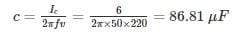Test: Power Systems- 4 - Question 8

A single – phase inductive load is drawing 10 MW at 0.6 power factor lagging. A capacitor is added in parallel across the load to improve the power factor to 0.85. What is the reactive power required for the capacitor?

Detailed Solution for Test: Power Systems- 4 - Question 8

Initially when the real power is 10 MW and power factor is 0.6, the reactive power is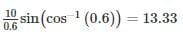Now when the capacitor is added, improved power factor is

cos-1 0.85 = 31.79°

Reactive power is 10 tan 31.79° = 6.2 VAR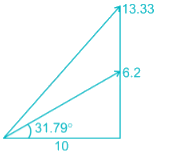Reactive power needed for the capacitor

= Qc = - (13.33 – 6.2)

= - 7.13 MVAR

*Answer can only contain numeric values
Test: Power Systems- 4 - Question 9

A single phase motor connected to 400V, 50Hz supply takes 31.7 A at a power factor of 0.7 lagging. Calculate the capacitance (in μF) required in parallel with the motor to raise the power factor to 0.9 lagging.

Detailed Solution for Test: Power Systems- 4 - Question 9

Active component of IM = IM cosϕM = 31.7 × 0.7 = 22.19 A

Active component of I = I cosϕ = I × 0.9 = 0.9I A

These components are represented by OA

0.9I = 22.19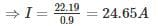Reactive component of IM = IM sinϕM =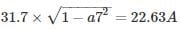Reactive component of I = I sinϕ =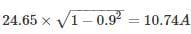Now, IC = Reactive component of IM – Reactive component of I

= 22.63 – 10.74 = 11.88 A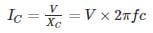⇒ 11.88 = 400 × 2π × 50 × c

⇒ c = 94.58 μF

Test: Power Systems- 4 - Question 10

A supply system feeds the following loads

(i) A lighting load of 500 kW

(ii) A load of 400 kW at a p.f. 0.707 lagging

(iv) A load of 500 kW at a p.f. 0.6 lagging

(v) A synchronous motor driving a 540 kW d.c. generator and having an overall efficiency of 90%

Calculate the power factor of the synchronous motor so that station power factor may become unity.

Detailed Solution for Test: Power Systems- 4 - Question 10

(i) The light load works at unity power factor.

P1 = 500 kW, Q1 = 0 KVAR

(ii) P2 = 400 kW, cosϕ2 = 0.707 lagging

Q2 = P tanϕ2 = 400 × tan cos-1 (0.707) = 400 KVAR (lagging)

(iii) P3 = 800 kW, cosϕ3 = 0.8 leadingQ3 = P3 tanϕ3 = 800 × tan cos-1(0.8) = 600 KVAR (leading)

(iv) P4 = 500 kW, cosϕ4 = 0.6 lagging

Q4 = P4 tanϕ4 = 500 × tan cos-1(0.6) = 666.67 KVAR (lagging)

Leading KVAR to be taken by synchronous motor

Q5 = Q4 + Q2 – Q3

Q5 = 400 + 666.67 – 600 = 466.66 KVAR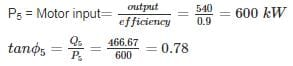## GATE Electrical Engineering (EE) 2023 Mock Test Series

22 docs|274 tests
Information about Test: Power Systems- 4 Page
In this test you can find the Exam questions for Test: Power Systems- 4 solved & explained in the simplest way possible. Besides giving Questions and answers for Test: Power Systems- 4, EduRev gives you an ample number of Online tests for practice

## GATE Electrical Engineering (EE) 2023 Mock Test Series

22 docs|274 tests(Scan QR code)